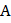# Five equal resistances each of resistanceare connected as shown in the figure. A battery ofvolts is connected betweenandThe current flowing inwill bea)b)c)d)## Question ID - 151297 :- Five equal resistances each of resistanceare connected as shown in the figure. A battery ofvolts is connected betweenandThe current flowing inwill bea)b)c)d)3537

(b)

The given circuit can be redrawn as followsEquivalent resistance betweenandisand currentNext Question :
 The voltage gain of a triode depends upon a) Filament voltage b) Plate voltage c) Plate resistance d) Plate current